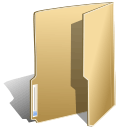## Chart (44)

Friday, 16 August 2019 01:05

### Create Pivot Chart in Excel in C#

Written by support iceblue

Starting from version 9.8.5, Spire.XLS supports creating pivot chart based on pivot table. This article is going to demonstrate how we can use Spire.XLS to implement this feature.

The input.xlsx Excel file: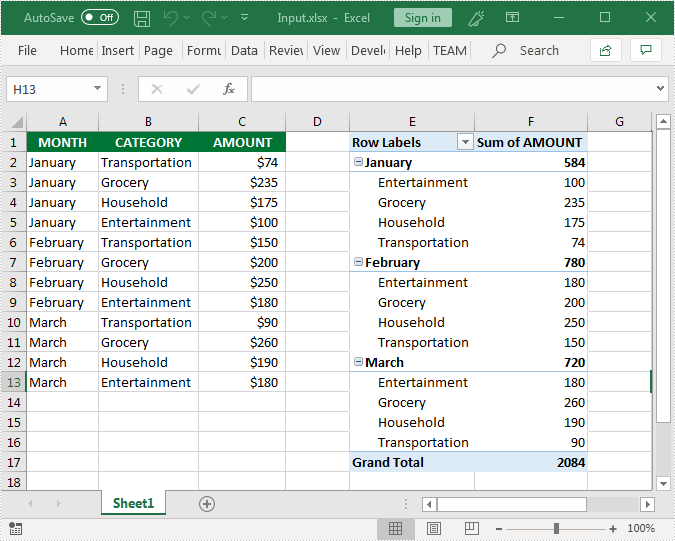Sample Code

```using Spire.Xls;
using Spire.Xls.Core;

namespace CreatePivotChart
{
class Program
{
static void Main(string[] args)
{
Workbook workbook = new Workbook();

//get the first worksheet
Worksheet sheet = workbook.Worksheets;
//get the first pivot table in the worksheet
IPivotTable pivotTable = sheet.PivotTables;

//create a clustered column chart based on the pivot table
//set chart position
chart.TopRow = 19;
chart.BottomRow = 38;
//set chart title
chart.ChartTitle = "Pivot Chart";

//save the resultant file
workbook.SaveToFile("CreatPivotChart.xlsx", ExcelVersion.Version2013);
}
}
}
```

Screenshot of the created pivot chart: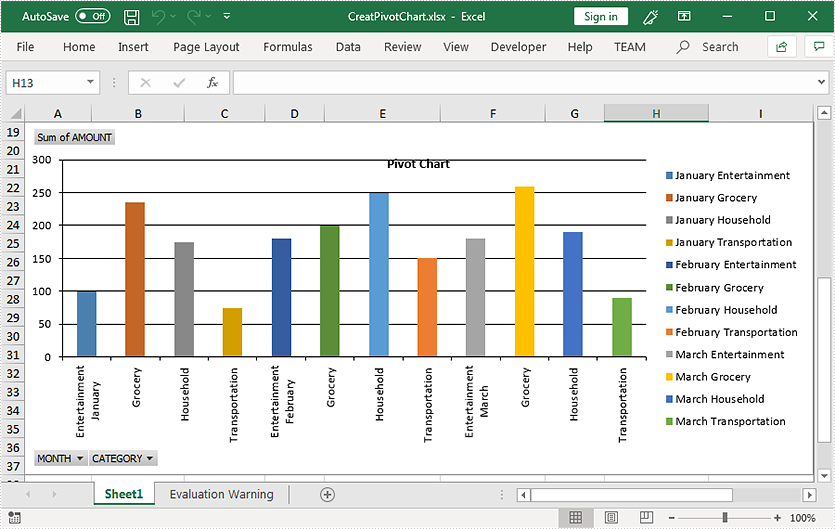Friday, 15 February 2019 08:27

### Create Chart without Using Worksheet Data Range in C#

Written by support iceblue

This article demonstrates how to create a chart without reference to the worksheet data range using Spire.XLS.

Detail steps:

Step 1: Create a workbook and get the first worksheet.

```Workbook wb = new Workbook();
Worksheet sheet = wb.Worksheets;
```

Step 2: Add a chart to the worksheet.

```Chart chart = sheet.Charts.Add();
```

Step 3: Add a series to the chart.

```var series = chart.Series.Add();
```

```series.EnteredDirectlyValues = new object[] { 10, 20, 30 };
```

Step 5: Save the file.

```wb.SaveToFile("result.xlsx", ExcelVersion.Version2013);
```

Output: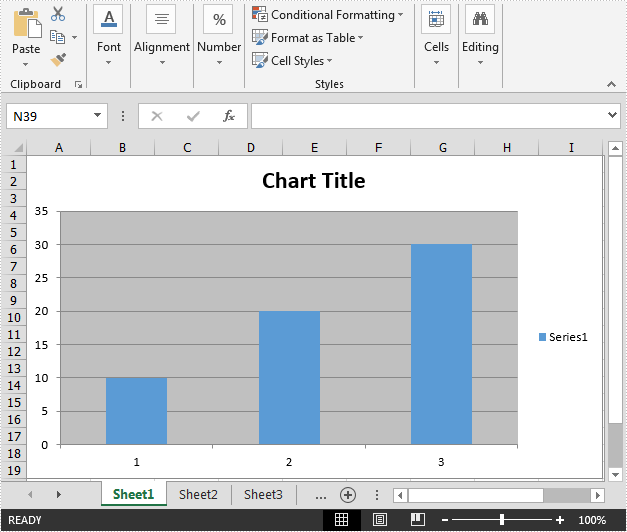Full code:

```using Spire.Xls;

namespace Create_chart
{
class Program
{
static void Main(string[] args)
{
//Create a workbook
Workbook wb = new Workbook();

//Get the first worksheet
Worksheet sheet = wb.Worksheets;

//Add a chart to the worksheet

//Add a series to the chart

series.EnteredDirectlyValues = new object[] { 10, 20, 30 };

//Save the file
wb.SaveToFile("result.xlsx", ExcelVersion.Version2013);
}
}
}
```
Thursday, 19 July 2018 09:26

### Apply Soft Edges effect to Excel Chart in C#

Written by support iceblue

This article elaborates the steps to apply soft edges effect to an excel chart using Spire.XLS.

The example Excel file we used for demonstration: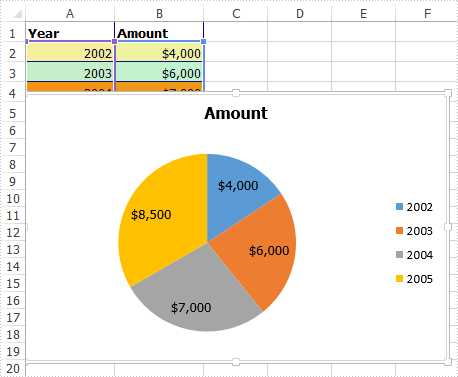Detail steps:

Step 1: Instantiate a Workbook object and load the excel file.

```Workbook workbook = new Workbook();
```

Step 2: Get the first worksheet.

```Worksheet sheet = workbook.Worksheets;
```

Step 3: Get the chart.

```IChart chart = sheet.Charts;
```

Step 4: Specify the size of the soft edge. Value can be set from 0 to 100.

```chart.ChartArea.Shadow.SoftEdge = 10;
```

Step 5: Save the file.

```workbook.SaveToFile("Output.xlsx", ExcelVersion.Version2013);
```

Output: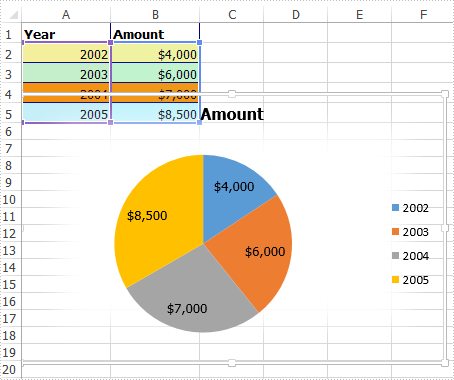Full code:

```using Spire.Xls;
using Spire.Xls.Core;

namespace Soft_Edges_in_Excel_Chart
{
class Program
{
static void Main(string[] args)
{
//Instantiate a Workbook object
Workbook workbook = new Workbook();

//Get the first worksheet
Worksheet sheet = workbook.Worksheets;

//Get the chart
IChart chart = sheet.Charts;

//Specify the size of the soft edge. Value can be set from 0 to 100

//Save the file
workbook.SaveToFile("Output.xlsx", ExcelVersion.Version2013);
}
}
}
```
Monday, 12 March 2018 07:55

### Delete Legend and Specific Legend Entries from Excel Chart in C#

Written by support iceblue

A legend is displayed in the chart area by default. However it can be removed from the chart. With Spire.XLS, we can delete the whole legend as well as specific legend entries from Excel chart. This article is going to demonstrate how we can use Spire.XLS to accomplish this function.

Below screenshot shows the Excel chart we used for demonstration: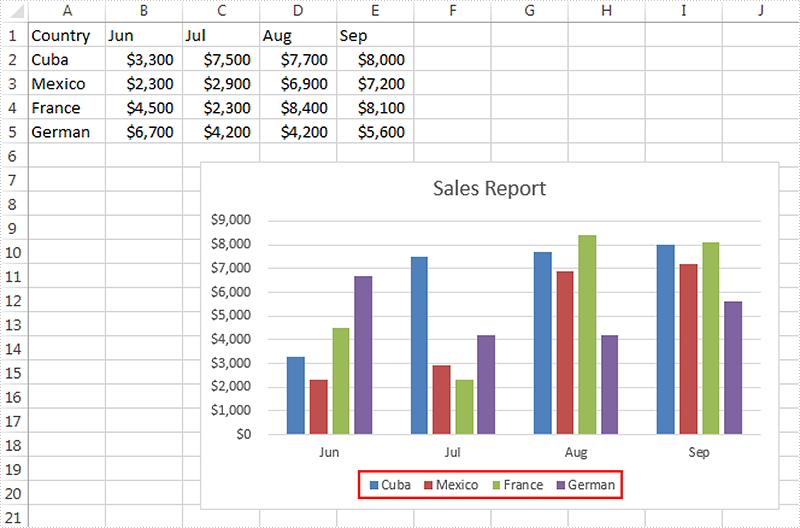Delete the whole legend

```//Create a Workbook instance
Workbook workbook = new Workbook();

//Get the first worksheet
Worksheet sheet = workbook.Worksheets;

//Get the chart
Chart chart = sheet.Charts;

//Delete legend from the chart
chart.Legend.Delete();

//Save the file
workbook.SaveToFile("DeleteLegend.xlsx", ExcelVersion.Version2013);
```

Screenshot: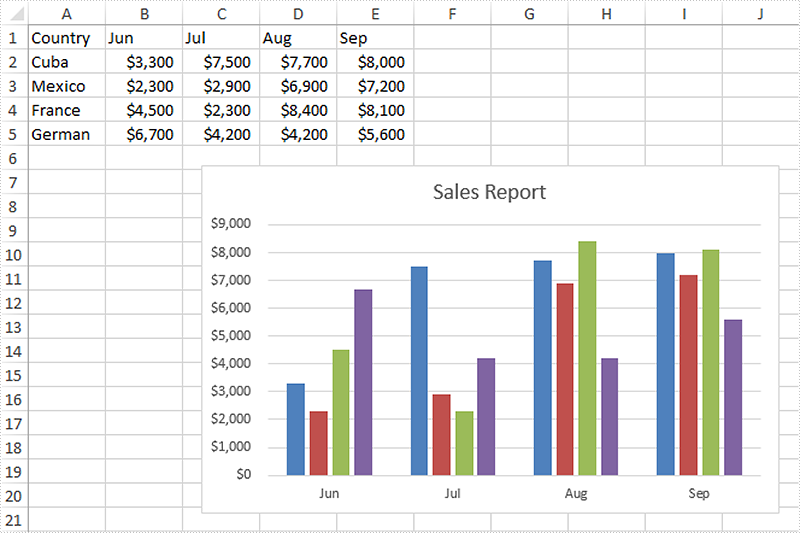Delete specific legend entries

```//Create a Workbook instance
Workbook workbook = new Workbook();

//Get the first worksheet
Worksheet sheet = workbook.Worksheets;

//Get the chart
Chart chart = sheet.Charts;

//Delete the first and the second legend entries from the chart
chart.Legend.LegendEntries.Delete();
chart.Legend.LegendEntries.Delete();

//Save the file
workbook.SaveToFile("DeleteLegendEntries.xlsx", ExcelVersion.Version2013);
```

Screenshot: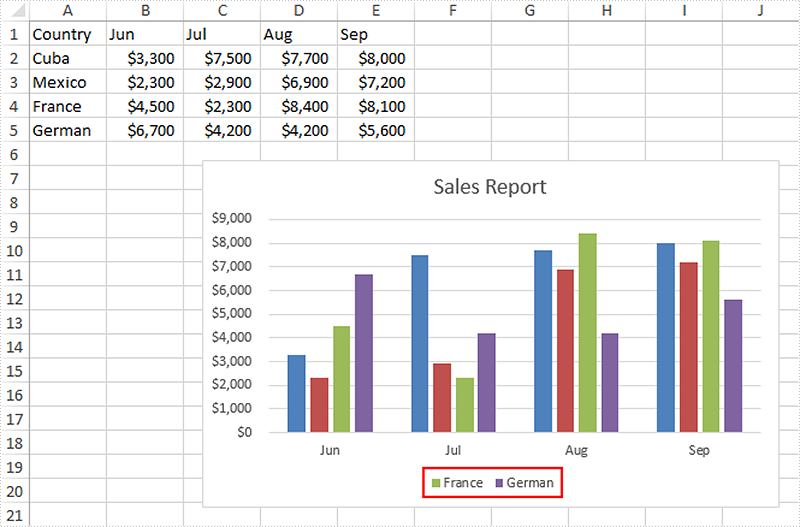Monday, 12 March 2018 07:12

### Create Multi-Level Category Chart in Excel in C#, VB.NET

Written by support iceblue

Multi-level category chart is a chart type that has both main category and subcategory labels. This type of chart is useful when you have figures for items that belong to different categories. In this article, you will learn how to create a multi-level category chart in Excel using Spire.XLS with C# and VB.NET.

Step 1: Create a Workbook instance and get the first worksheet.

```Workbook wb = new Workbook();
Worksheet sheet = wb.Worksheets;
```

Step 2: Write data to cells.

```sheet.Range["A1"].Text = "Main Category";
sheet.Range["A2"].Text = "Fruit";
sheet.Range["A6"].Text = "Vegies";
sheet.Range["B1"].Text = "Sub Category";
sheet.Range["B2"].Text = "Bananas";
sheet.Range["B3"].Text = "Oranges";
sheet.Range["B4"].Text = "Pears";
sheet.Range["B5"].Text = "Grapes";
sheet.Range["B6"].Text = "Carrots";
sheet.Range["B7"].Text = "Potatoes";
sheet.Range["B8"].Text = "Celery";
sheet.Range["B9"].Text = "Onions";
sheet.Range["C1"].Text = "Value";
sheet.Range["C2"].Value = "52";
sheet.Range["C3"].Value = "65";
sheet.Range["C4"].Value = "50";
sheet.Range["C5"].Value = "45";
sheet.Range["C6"].Value = "64";
sheet.Range["C7"].Value = "62";
sheet.Range["C8"].Value = "89";
sheet.Range["C9"].Value = "57";
```

Step 3: Vertically merge cells from A2 to A5, A6 to A9.

```sheet.Range["A2:A5"].Merge();
sheet.Range["A6:A9"].Merge();
```

Step 4: Add a clustered bar chart to worksheet.

```Chart chart = sheet.Charts.Add(ExcelChartType.BarClustered);
chart.ChartTitle = "Value";
chart.PlotArea.Fill.FillType = ShapeFillType.NoFill;
chart.Legend.Delete();
```

Step 5: Set the data source of series data.

```chart.DataRange = sheet.Range["C2:C9"];
chart.SeriesDataFromRange = false;
```

Step 6: Set the data source of category labels.

```ChartSerie serie = chart.Series;
serie.CategoryLabels = sheet.Range["A2:B9"];
```

Step 7: Show multi-level category labels.

```chart.PrimaryCategoryAxis.MultiLevelLable = true;
```

Step 8: Save the file.

```wb.SaveToFile("output.xlsx", ExcelVersion.Version2013);
```

Output: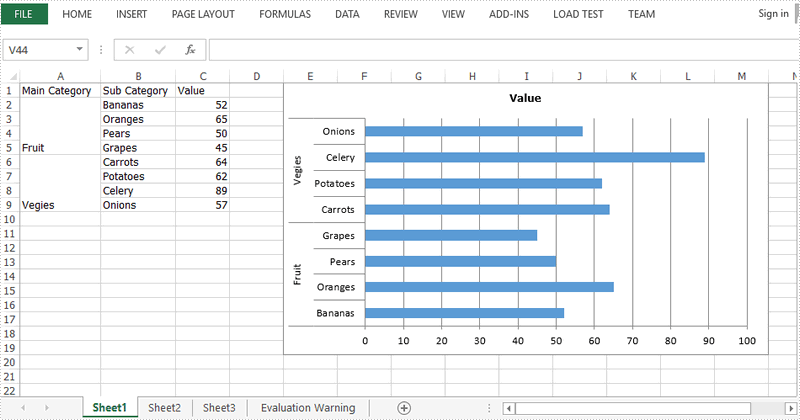Full Code:

[C#]
```Workbook wb = new Workbook();
Worksheet sheet = wb.Worksheets;

sheet.Range["A1"].Text = "Main Category";
sheet.Range["A2"].Text = "Fruit";
sheet.Range["A6"].Text = "Vegies";
sheet.Range["B1"].Text = "Sub Category";
sheet.Range["B2"].Text = "Bananas";
sheet.Range["B3"].Text = "Oranges";
sheet.Range["B4"].Text = "Pears";
sheet.Range["B5"].Text = "Grapes";
sheet.Range["B6"].Text = "Carrots";
sheet.Range["B7"].Text = "Potatoes";
sheet.Range["B8"].Text = "Celery";
sheet.Range["B9"].Text = "Onions";
sheet.Range["C1"].Text = "Value";
sheet.Range["C2"].Value = "52";
sheet.Range["C3"].Value = "65";
sheet.Range["C4"].Value = "50";
sheet.Range["C5"].Value = "45";
sheet.Range["C6"].Value = "64";
sheet.Range["C7"].Value = "62";
sheet.Range["C8"].Value = "89";
sheet.Range["C9"].Value = "57";
sheet.Range["A2:A5"].Merge();
sheet.Range["A6:A9"].Merge();
sheet.AutoFitColumn(1);
sheet.AutoFitColumn(2);

chart.ChartTitle = "Value";
chart.PlotArea.Fill.FillType = ShapeFillType.NoFill;
chart.Legend.Delete();
chart.LeftColumn = 5;
chart.TopRow = 1;
chart.RightColumn = 14;
chart.DataRange = sheet.Range["C2:C9"];
chart.SeriesDataFromRange = false;
ChartSerie serie = chart.Series;
serie.CategoryLabels = sheet.Range["A2:B9"];
chart.PrimaryCategoryAxis.MultiLevelLable = true;
wb.SaveToFile("output.xlsx", ExcelVersion.Version2013);
```
[VB.NET]
```Dim wb As Workbook = New Workbook()
Dim sheet As Worksheet = wb.Worksheets(0)

sheet.Range("A1").Text = "Main Category"
sheet.Range("A2").Text = "Fruit"
sheet.Range("A6").Text = "Vegies"
sheet.Range("B1").Text = "Sub Category"
sheet.Range("B2").Text = "Bananas"
sheet.Range("B3").Text = "Oranges"
sheet.Range("B4").Text = "Pears"
sheet.Range("B5").Text = "Grapes"
sheet.Range("B6").Text = "Carrots"
sheet.Range("B7").Text = "Potatoes"
sheet.Range("B8").Text = "Celery"
sheet.Range("B9").Text = "Onions"
sheet.Range("C1").Text = "Value"
sheet.Range("C2").Value = "52"
sheet.Range("C3").Value = "65"
sheet.Range("C4").Value = "50"
sheet.Range("C5").Value = "45"
sheet.Range("C6").Value = "64"
sheet.Range("C7").Value = "62"
sheet.Range("C8").Value = "89"
sheet.Range("C9").Value = "57"
sheet.Range("A2:A5").Merge()
sheet.Range("A6:A9").Merge()
sheet.AutoFitColumn(1)
sheet.AutoFitColumn(2)

Dim chart As Chart = sheet.Charts.Add(ExcelChartType.BarClustered)
chart.ChartTitle = "Value"
chart.PlotArea.Fill.FillType = ShapeFillType.NoFill
chart.Legend.Delete()
chart.LeftColumn = 5
chart.TopRow = 1
chart.RightColumn = 14
chart.DataRange = sheet.Range("C2:C9")
chart.SeriesDataFromRange = False
Dim serie As ChartSerie =  chart.Series(0)
serie.CategoryLabels = sheet.Range("A2:B9")
chart.PrimaryCategoryAxis.MultiLevelLable = True
wb.SaveToFile("output.xlsx", ExcelVersion.Version2013)
```
Tuesday, 27 February 2018 07:37

### How to set the rotation for the 3D chart on Excel in C#

Written by support iceblue

With Spire.XLS, we can set the 3-D rotation easily in C#. The following code example explains how to set the rotation of the 3D chart view in C#. We will use 3D pie chart for example. Firstly, view the original 3D pie chart: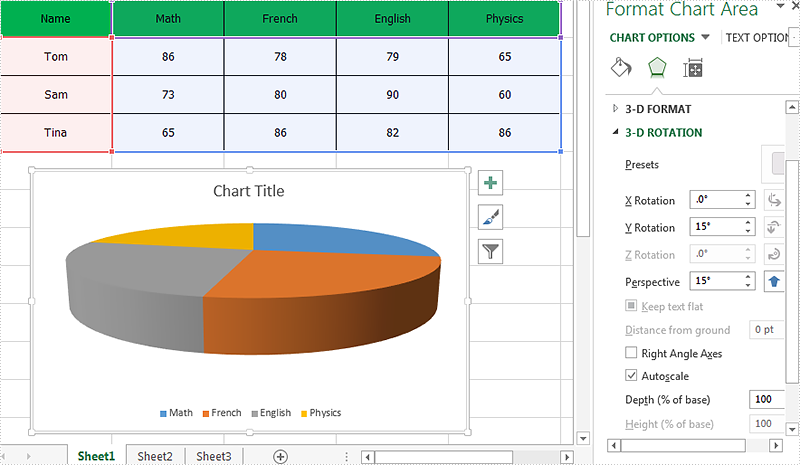Code snippet of how to set the 3D rotation for Excel Chart:

Step 1: Create a new instance of workbook and load the sample document from file.

```Workbook workbook = new Workbook();
```

Step 2: Get the chart from the first worksheet.

```Worksheet sheet = workbook.Worksheets;
Chart chart = sheet.Charts;
```

Step 3: Set Rotation of the 3D chart view for X and Y.

```//X rotation:
chart.Rotation=30;
//Y rotation:
chart.Elevation = 20;
```

Step 4: Save the document to file.

```workbook.SaveToFile("Result.xlsx", ExcelVersion.Version2010);
```

Effective screenshot of the Excel 3D chart rotation: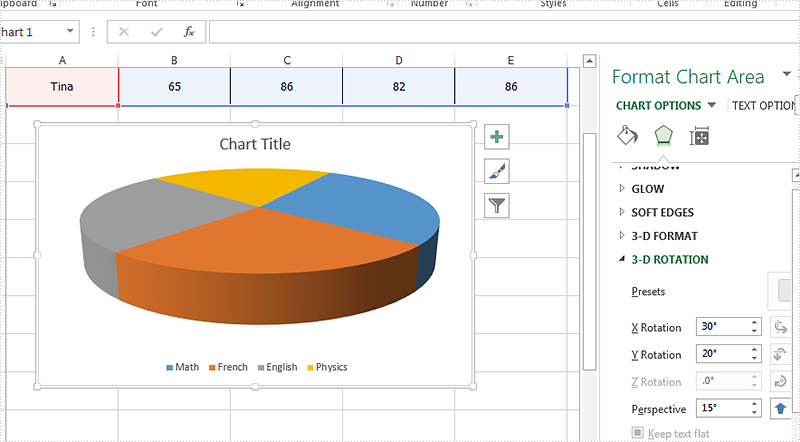Full codes:

```Workbook workbook = new Workbook();

Worksheet sheet = workbook.Worksheets;
Chart chart = sheet.Charts;

//X rotation:
chart.Rotation=30;
//Y rotation:
chart.Elevation = 20;

workbook.SaveToFile("Result.xlsx", ExcelVersion.Version2010);
```
Tuesday, 06 February 2018 07:37

### How to explode a doughnut chart in C#

Written by support iceblue

In the previous article, we have demonstrated how to explode a pie chart via Spire.XLS. This article we will explain how to explode a doughnut chart in C#.

When we need to explode a doughnut chart, we can set the chart type as DoughnutExploded when we create a doughnut chart from scratch. For the existing doughnut chart, we can easily change the chart type to explode the doughnut chart.

Step 1: Create an instance of Excel workbook and load the document from file.

```Workbook workbook = new Workbook();
```

Step 2: Get the first worksheet from the sample workbook.

```Worksheet sheet = workbook.Worksheets;
```

Step 3: Get the first chart from the first worksheet.

```Chart chart = sheet.Charts;
```

Step 4: Set the chart type as DoughnutExploded to explode the doughnut chart.

```chart.ChartType = ExcelChartType.DoughnutExploded;
```

Step 5: Save the document to file.

```workbook.SaveToFile("ExplodedDoughnutChart.xlsx", ExcelVersion.Version2010);
```

Effective screenshot of the explode doughnut chart: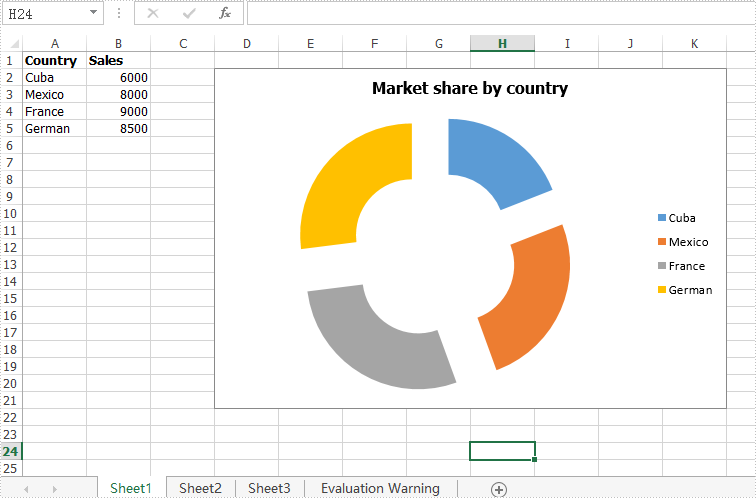Full codes of how to explode a doughnut chart:

```Workbook workbook = new Workbook();

Worksheet sheet = workbook.Worksheets;
Chart chart = sheet.Charts;
chart.ChartType = ExcelChartType.DoughnutExploded;

workbook.SaveToFile("ExplodedDoughnutChart.xlsx", ExcelVersion.Version2010);
```
Wednesday, 13 September 2017 09:21

### How to remove chart from Excel worksheet in C#, VB.NET

Written by support iceblue

With Spire.XLS for .NET, we could easily add different kinds of charts to Excel worksheet, such as Pie Chart, Column Chart, bar chart, line chart, radar chart, Doughnut chart, pyramid chart, etc. In this article, we'll show you how to remove chart from Excel worksheet by using Spire.XLS.

Below is a sample document which contains a chart and table from the Excel worksheet, then we'll remove the chart from the slide.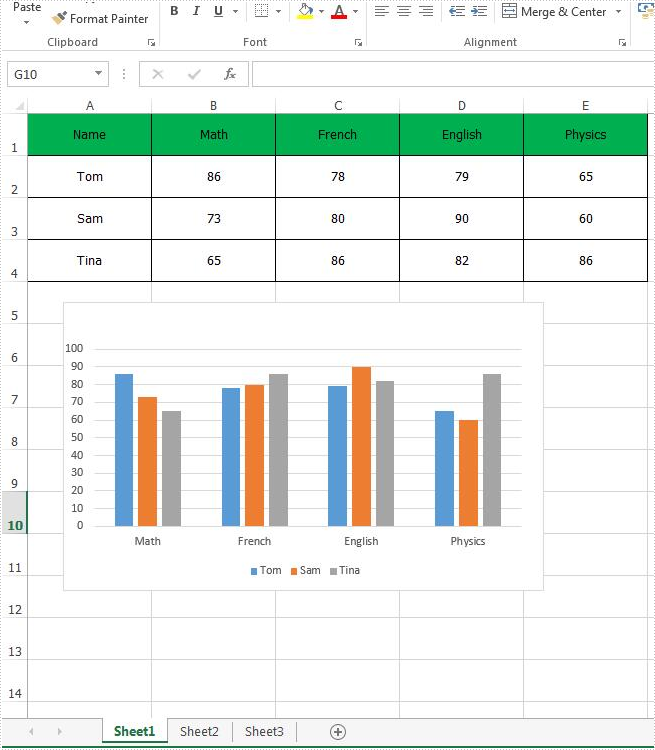C# Code Snippet of how to remove the chart from Excel:

Step 1: Create an instance of Excel workbook and load the document from file.

```Workbook workbook = new Workbook();
```

Step 2: Get the first worksheet from the workbook.

```Worksheet sheet = workbook.Worksheets;
```

Step 3: Get the first chart from the first worksheet.

```IChartShape chart = sheet.Charts;
```

Step 4: Remove the chart.

```chart.Remove();
```

Step 5: Save the document to file.

```workbook.SaveToFile("RemoveChart.xlsx");
```

Effective screenshot: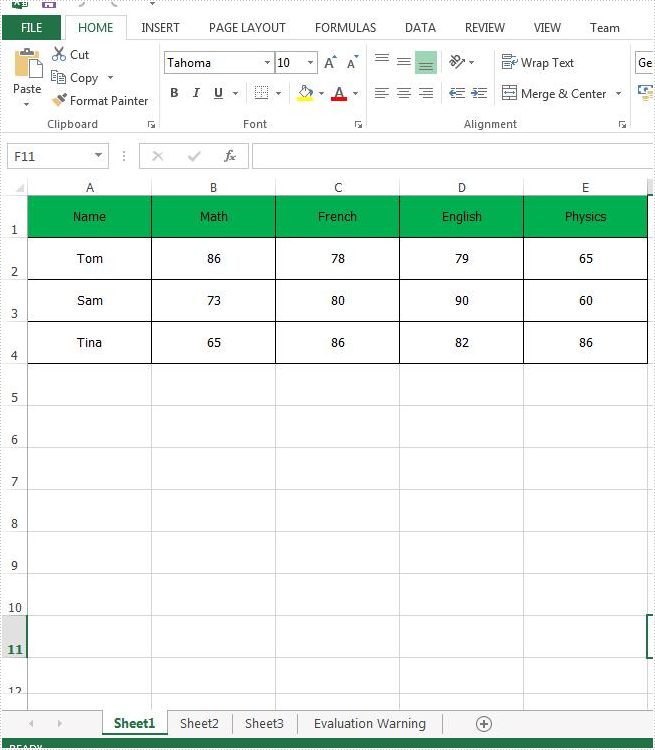Full code:

[C#]
```Workbook workbook = new Workbook();
Worksheet sheet = workbook.Worksheets;
IChartShape chart = sheet.Charts;
chart.Remove();
workbook.SaveToFile("RemoveChart.xlsx");
```
[VB.NET]
```Dim workbook As New Workbook()
Dim sheet As Worksheet = workbook.Worksheets(0)
Dim chart As IChartShape = sheet.Charts(0)
chart.Remove()
workbook.SaveToFile("RemoveChart.xlsx")
```
Friday, 16 December 2016 07:11

### How to Create a Doughnut Chart in Excel in C#

Written by support iceblue

A donut chart is a variant of the pie chart, with a blank center allowing for additional information about the data as a whole to be included. In this article, you will learn how to create a doughnut chart using Spire.XLS in C#.

Step 1: Initialize a new instance of Workbook class and set the Excel version as 2013.

```Workbook wb = new Workbook();
wb.Version = ExcelVersion.Version2013;
```

Step 2: Get the first sheet from workbook.

```Worksheet sheet = wb.Worksheets;
```

Step 3: Insert some data in the sheet.

```sheet.Range["A1"].Value = "Country";
sheet.Range["A1"].Style.Font.IsBold = true;
sheet.Range["A2"].Value = "Cuba";
sheet.Range["A3"].Value = "Mexico";
sheet.Range["A4"].Value = "France";
sheet.Range["A5"].Value = "German";
sheet.Range["B1"].Value = "Sales";
sheet.Range["B1"].Style.Font.IsBold = true;
sheet.Range["B2"].NumberValue = 6000;
sheet.Range["B3"].NumberValue = 8000;
sheet.Range["B4"].NumberValue = 9000;
sheet.Range["B5"].NumberValue = 8500;
```

Step 4: Create a Doughnut Chart based on the data from range A1:B5.

```Chart chart = sheet.Charts.Add();
chart.ChartType = ExcelChartType.Doughnut;
chart.DataRange = sheet.Range["A1:B5"];
chart.SeriesDataFromRange = false;
```

Step 5: Set the chart position.

```chart.LeftColumn = 4;
chart.TopRow = 2;
chart.RightColumn = 12;
chart.BottomRow = 22;
```

Step 6: Display percentage value in data labels.

```foreach (ChartSerie cs in chart.Series)
{
cs.DataPoints.DefaultDataPoint.DataLabels.HasPercentage = true;
}
```

Step 7: Save the file.

```wb.SaveToFile("DoughnutChart.xlsx",ExcelVersion.Version2010);
```

Output: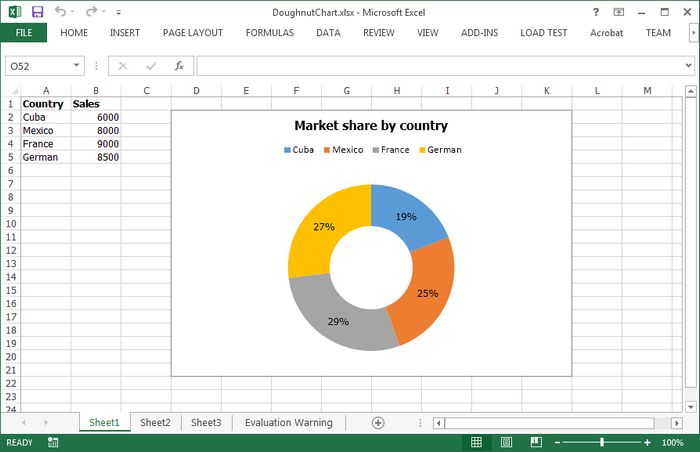Full Code:

```using Spire.Xls;
using Spire.Xls.Charts;

namespace DoughnutChart
{
class Program
{
static void Main(string[] args)
{
Workbook wb = new Workbook();
wb.Version = ExcelVersion.Version2013;
Worksheet sheet = wb.Worksheets;

//insert data
sheet.Range["A1"].Value = "Country";
sheet.Range["A1"].Style.Font.IsBold = true;
sheet.Range["A2"].Value = "Cuba";
sheet.Range["A3"].Value = "Mexico";
sheet.Range["A4"].Value = "France";
sheet.Range["A5"].Value = "German";
sheet.Range["B1"].Value = "Sales";
sheet.Range["B1"].Style.Font.IsBold = true;
sheet.Range["B2"].NumberValue = 6000;
sheet.Range["B3"].NumberValue = 8000;
sheet.Range["B4"].NumberValue = 9000;
sheet.Range["B5"].NumberValue = 8500;

//add a new chart, set chart type as doughnut
chart.ChartType = ExcelChartType.Doughnut;
chart.DataRange = sheet.Range["A1:B5"];
chart.SeriesDataFromRange = false;

//set position of chart
chart.LeftColumn = 4;
chart.TopRow = 2;
chart.RightColumn = 12;
chart.BottomRow = 22;

//chart title
chart.ChartTitle = "Market share by country";
chart.ChartTitleArea.IsBold = true;
chart.ChartTitleArea.Size = 12;

foreach (ChartSerie cs in chart.Series)
{
cs.DataPoints.DefaultDataPoint.DataLabels.HasPercentage = true;
}

chart.Legend.Position = LegendPositionType.Top;
wb.SaveToFile("DoughnutChart.xlsx",ExcelVersion.Version2010);
}
}
}
```
Thursday, 15 December 2016 07:36

### Set font for the text on Chart title and Chart Axis in C#

Written by support iceblue

Spire.XLS offers multiple functions to enable developers to set the font for the text for Excel chart. We have already demonstrated how to set the font for the text on legend and datalable in Excel chart by using the SetFont() in C#. This article will focus on showing how to set font for the text on Chart title and Chart Axis.

Firstly, please view the Excel worksheet with chart which the font will be changed later: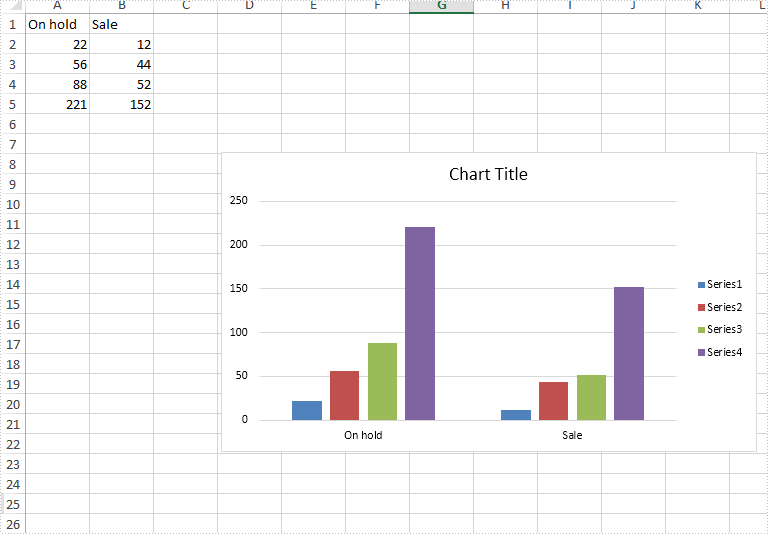Step 1: Create a new Excel workbook and load from file.

```Workbook workbook = new Workbook();
```

Step 2: Get the first worksheet from workbook.

```Worksheet worksheet = workbook.Worksheets;
Spire.Xls.Chart chart = worksheet.Charts;
```

Step 3: Format the font for the chart title.

```chart.ChartTitleArea.Font.Color = Color.Blue;
chart.ChartTitleArea.Font.Size = 20.0;
```

Step 4: Format the font for the chart Axis.

```chart.PrimaryValueAxis.Font.Color = Color.Gold;
chart.PrimaryValueAxis.Font.Size = 10.0;

chart.PrimaryCategoryAxis.Font.Color = Color.Red;
chart.PrimaryCategoryAxis.Font.Size = 20.0;
```

Step 5: Save the document to file.

```workbook.SaveToFile("result.xlsx", FileFormat.Version2010);
```

Effective screenshot after formatting the font for the chart title and chart axis.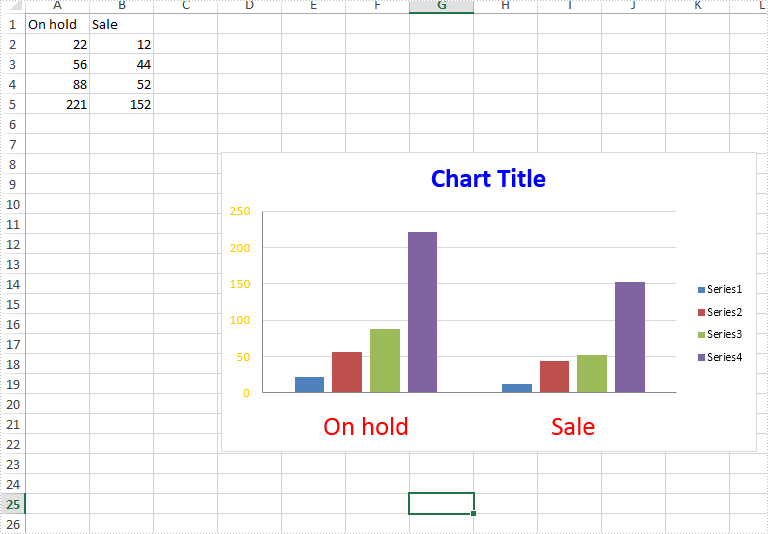Full codes:

```static void Main(string[] args)
{
Workbook workbook = new Workbook();

Worksheet worksheet = workbook.Worksheets;
Spire.Xls.Chart chart = worksheet.Charts;

chart.ChartTitleArea.Font.Color = Color.Blue;
chart.ChartTitleArea.Font.Size = 20.0;

chart.PrimaryValueAxis.Font.Color = Color.Gold;
chart.PrimaryValueAxis.Font.Size = 10.0;

chart.PrimaryCategoryAxis.Font.Color = Color.Red;
chart.PrimaryCategoryAxis.Font.Size = 20.0;

workbook.SaveToFile("result.xlsx", FileFormat.Version2010);

}
```
Page 1 of 4